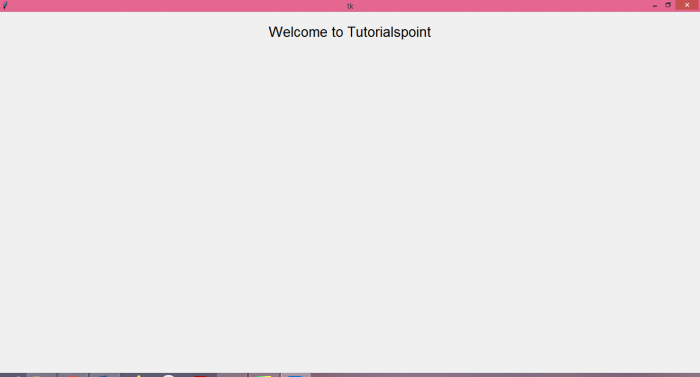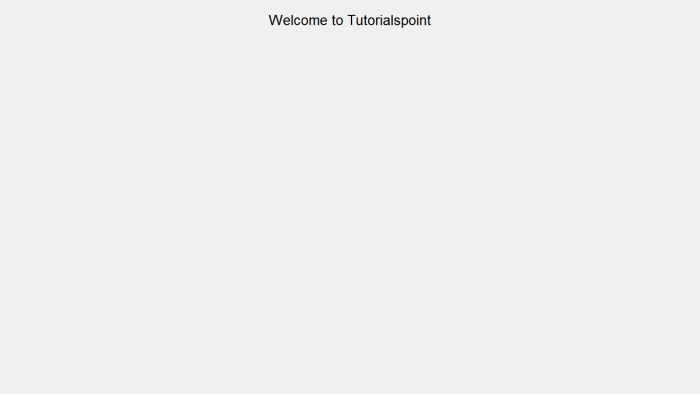# Creating an automatically maximized tkinter window

TkinterPythonGUI-Programming

There are two different ways in which we can get an automatically maximized window in Tkinter.

• We can use the state() method of Tkinter and invoke it with the attribute "zoomed".
root.state("zoomed")
• The second approach is to use the attributes method of Tkinter with the parameter "-fullscreen" and set it to True.

By default, Tkinter creates a window of a predefined size. The dimensions of the window can be customized using the geometry method. For example,

root.geometry("700 x 350")


## Example 1

# Import the required libraries
from tkinter import *

# Create an instance of tkinter frame
root=Tk()

# Create a label
Label(root, text="Welcome to Tutorialspoint", font="Calibri, 20").pack(pady=20)

# Maximize the window Size using state property
root.state('zoomed')

root.mainloop()

## Output

It will produce the following output −## Example 2

Now, let's tweak the code and use the attribute method instead of the state method.

# Import the required libraries
from tkinter import *

# Create an instance of tkinter frame
root=Tk()

# Create a label
Label(root, text="Welcome to Tutorialspoint", font="Calibri, 20").pack(pady=20)

# Maximize the window Size using attributes method
root.attributes('-fullscreen', True)

root.mainloop()

## Output

It will produce the following output −